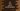# C# program to find the sum of all odd numbers below a given number## C# find the sum of all odd numbers below a given number:

In this post, we will learn how to find the sum of all odd numbers below a given number. A number is called odd if it is not divisible by 2. This can be solved by using one loop and iterate from 1 to that number, check if the number is odd and add it to a sum variable. To find the sum, we need to create a sum variable initializing it as 0. We can add all odd numbers to this sum variable.

### Method 1: Find the sum of all odd numbers in C# using a for loop:

Let’s try to solve it first by using a for loop. Let’s take a look at the below program:

``````using System;

public class Program {

public static void Main(string[] args) {
int i, limit, sumOdd = 0;
Console.WriteLine("Enter the limit :");

for(i = 1; i<= limit; i++){
if(i % 2 != 0){
sumOdd += i;
}
}

Console.WriteLine("Total sum: "+sumOdd);
}
}``````

Here,

• If you run the program, the Main method is called first. The first is initializing three variables: i, limit and sumOdd. i is to use in the for loop, limit to store the maximum number which is entered by the user and sumOdd is to keep the sum of all odd values from 1 to limit.
• The second and third lines are used to ask the user to enter a number for the limie and to read and store that value in the variable limit.
• We are running a for loop that runs from 1 to limit. For each value it finds, it is checking if that is divisible by 2 or not. If it is not divisible by 2, i.e. if it is odd, we are adding that value to sumOdd.
• Finally, the last line prints the value of sum of all odd numbers from 1 to limit.

If you run this program, it will give output as like below:

``````Enter the limit :
10
Total sum: 25``````

This sum is calculated by adding 1 + 3 + 5 + 7 + 9.

### Method 2: By incrementing 2 in the for loop:

This is an improvement of the above method. We can start from 1 and increment by 2 on each step in the for loop. Also, we don’t have to check for each value if it is odd or not. Because, if we increment by 2 starting from 1, all values will be odd like 1, 3, 5, 7, 9, 11 … etc.

The program will be as like below:

``````using System;

public class Program {

public static void Main(string[] args) {
int i, limit, sumOdd = 0;
Console.WriteLine("Enter the limit :");

for(i = 1; i<= limit; i+=2){
sumOdd += i;
}

Console.WriteLine("Total sum: "+sumOdd);
}
}``````

Here,

• The only difference we made is in the for loop. The value of i is increments by 2 on each iteration.
• The sumOdd is incremented without any check, which makes it one step lesser.

If you run this program, it will give similar results.

### Method 3: By using a while loop:

We can replace the for loop with any other loop. Let’s try with a while loop. This loop will use the same process we used in the second method above, i.e. it will starts from 1 and increment the value by 2.

``````using System;

public class Program {

public static void Main(string[] args) {
int i = 1, limit, sumOdd = 0;
Console.WriteLine("Enter the limit :");

while(i<=limit){
sumOdd += i;
i += 2;
}
Console.WriteLine("Total sum: "+sumOdd);
}
}``````

Here,

• The while loop starts from i = 1 and it ends on limit.
• Inside the loop, we are incrementing the value of i by 2 on each step.

If you run this program, it will give similar output.

``````Enter the limit :
100
Total sum: 2500``````